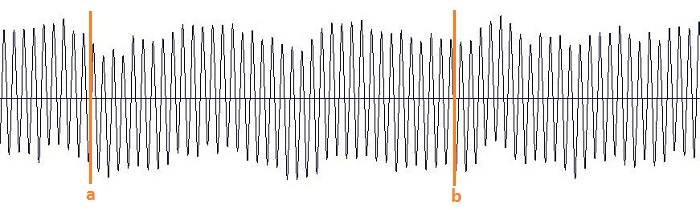#music
#photo
#opensource
#all

Don't read #tech articles except you really want to.

Some of my projects:
BigPicture
SamplerBox
Ojourdui

23/01/2021

## How to find seamless loops in audio files (with a little bit of math and programming)

When making instrument sample sets (e.g. church organ sample sets used with Hauptwerk or GrandOrgue), we need to set looping points in WAV audio files:such that when playing the part [a, b] in loop, we don't hear any click or pop when the sample reaches the end of the loop.

Example 1: bad loop with audible clicks

Example 2: seamless loop with no click, that's what we are looking for! The loop has a ~ 2.670 second period, can you hear where are the looping points?

Finding looping points can be done manually but this is a very long and tedious task. A few programs exist to do this process automatically such as Extreme Sample Converter (it has an excellent auto-looping algorithm), LoopAuditioneer (open source), Zero-X Seamless Looper, SampleLooper, etc.

Here we'll look at a home-cooked algorithm that works well to detect looping points.

``````from scipy.io import wavfile
import numpy as np
import itertools

x0 = x if x.ndim == 1 else x[:, 0]     # let's keep only 1 channel for simplicity, but we could easily generalize this for 2 channels
x0 = np.asarray(x0, dtype=np.float32)``````

Let's say the audio file's sustain part (this is precisely where we're looking for a loop!) begins at t=2 sec and finishes at t=9 sec. We will now subdivide the time-interval [2 sec, 9 sec] into a 250 milliseconds grid: 2, 2.25, 2.5, 2.75, 3, 3.25, ..., 8.75, 9.

From this sequence, we now create "loop candidates" (a, b) of length at least 1 second, example: (2.5, 7.5), (3.25, 5.75), (6.0, 8.75), etc. Then, for each loop candidate, we'll improve the loop (this is the core of the algorithm, it will be discussed in the next paragraph) and compute a distance `d`. We finally keep the loop that has the minimal distance (among all loop candidates). Finished!

``````A = [int((2 + 0.25 * k) * sr) for k in range(29)]  # the grid 2, 2.25, 2.5, ... 8.75, 9
dist = np.inf
for a, b in itertools.product(A, A):  # cartesian product: pairs (a, b) of points on the grid
if b - a < 1 * sr:
continue
a, B, d = improveloop(x0, a, b, sr=sr)
print 'Loop (%.3fs, %.3fs) improved to (%.3fs, %.3fs), distance: %i' % (a * 1.0 / sr, b * 1.0 / sr, a * 1.0 / sr, B * 1.0 / sr, d)

if d < dist:
aa = a
BB = B
dist = d

print "The final loop is (%.3fs, %.3fs), i.e. (%i, %i)." % (aa * 1.0 / sr, BB * 1.0 / sr, aa, BB)``````

Finished? Not yet! We need to explain what we mean by improving a loop, as that's the crucial part of the algorithm. More precisely, we'll now explain how to transform a loop (3.25, 5.75) with points taken on the grid (this random loop probably "clicks" like in Example 1 before!) into a "good loop" (3.25, 5.831). Let's zoom on the junction point to understand what's going on:

How to measure if a loop is good or not? Ideally, if the loop (a, b) is perfect/seamless, `x[a:a+10 ms]` should be very close to `x[b:b+10 ms]`. Measuring how close two arrays `x` and `y` are can be done by computing `sum((x[n]-y[n])^2)`, and if the sum is small, `x` and `y` are close.

Finding `k` such that `np.sum(np.abs(x0[a:a+W1]-x0[k+b:k+b+W1])**2)` is minimal can be obtained by noting that

``(x[n] - y[n+k])**2  = x[n]**2 - 2*x[n]*y[n+k] + y[n+k]**2``

and by using numpy.correlate. We can now define this function:

``````def improveloop(x0, a, b, sr=44100, w1=0.010, w2=0.100):
"""
Input:  (a, b) is a loop
Output: (a, B) is a better loop
distance (the less the distance the better the loop)
This function moves the loop's endpoint b to B (up to 100 ms further) such that (a, B) is a "better" loop, i.e. sum((x0[a:a+10ms] - x0[B:B+10ms])^2) is minimal
"""

W1 = int(w1*sr)
W2 = int(w2*sr)
x = x0[a:a+W1]
y = x0[b:b+W2]
delta = np.sum(x**2) - 2*np.correlate(y, x) + np.correlate(y**2, np.ones_like(x))
K = np.argmin(delta)
B = K + b
distance = delta[K]

return a, B, distance``````

That's it, in less than 50 lines of Python code!

This audio file

(looped 4 times here but we could loop it forever) has been obtained with the algorithm described here. Not too bad, n'est-ce pas?

Example of output:

``````Loop (2.000s, 3.000s) improved to (2.000s, 3.009s), distance: 1003724800
Loop (2.000s, 3.250s) improved to (2.000s, 3.340s), distance: 839278592
Loop (2.000s, 3.500s) improved to (2.000s, 3.559s), distance: 1281863680
[...]
Loop (2.000s, 8.500s) improved to (2.000s, 8.544s), distance: 1092337664
Loop (2.000s, 8.750s) improved to (2.000s, 8.789s), distance: 964747264
Loop (2.000s, 9.000s) improved to (2.000s, 9.004s), distance: 2488913920
[...]
Loop (7.750s, 9.000s) improved to (7.750s, 9.004s), distance: 1167093760
Loop (8.000s, 9.000s) improved to (8.000s, 9.001s), distance: 1710333952

The final loop is (6.750s, 8.322s), i.e. (297675, 366989).``````

Note: Wouldn't it be possible to save these loop markers inside the WAV file's metadata instead of just printing them on screen? Sure it is, but as Python's standard library doesn't support WAV markers editing, you'll have to use these techniques to do this.